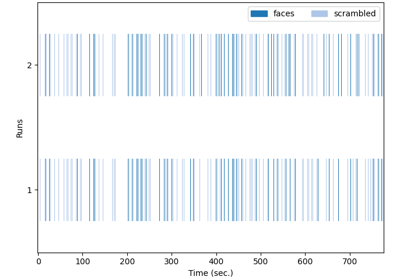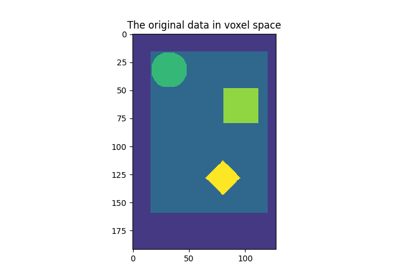Note

This page is a reference documentation. It only explains the function signature, and not how to use it. Please refer to the user guide for the big picture.

# nilearn.image.resample_img#

nilearn.image.resample_img(img, target_affine=None, target_shape=None, interpolation='continuous', copy=True, order='F', clip=True, fill_value=0, force_resample=False)[source]#

Resample a Niimg-like object.

Parameters:
imgNiimg-like object

See Input and output: neuroimaging data representation. Image(s) to resample.

target_affinenumpy.ndarray, optional

If specified, the image is resampled corresponding to this new affine. target_affine can be a 3x3 or a 4x4 matrix. (See notes)

target_shapetuple or list, optional

If specified, the image will be resized to match this new shape. len(target_shape) must be equal to 3. If target_shape is specified, a target_affine of shape (4, 4) must also be given. (See notes)

interpolationstr, optional

Can be ‘continuous’, ‘linear’, or ‘nearest’. Indicates the resample method. Default=’continuous’.

copybool, optional

If True, guarantees that output array has no memory in common with input array. In all cases, input images are never modified by this function. Default=True.

order“F” or “C”, optional

Data ordering in output array. This function is slightly faster with Fortran ordering. Default=’F’.

clipbool, optional

If True (default) all resampled image values above max(img) and under min(img) are clipped to min(img) and max(img). Note that 0 is added as an image value for clipping, and it is the padding value when extrapolating out of field of view. If False no clip is performed. Default=True.

fill_valuefloat, optional

Use a fill value for points outside of input volume. Default=0.

force_resamplebool, optional

Intended for testing, this prevents the use of a padding optimization. Default=False.

Returns:
resamplednibabel.Nifti1Image

input image, resampled to have respectively target_shape and target_affine as shape and affine.

Notes

BoundingBoxError If a 4x4 transformation matrix (target_affine) is given and all of the transformed data points have a negative voxel index along one of the axis, then none of the data will be visible in the transformed image and a BoundingBoxError will be raised.

If a 4x4 transformation matrix (target_affine) is given and no target shape is provided, the resulting image will have voxel coordinate (0, 0, 0) in the affine offset (4th column of target affine) and will extend far enough to contain all the visible data and a margin of one voxel.

3x3 transformation matrices If a 3x3 transformation matrix is given as target_affine, it will be assumed to represent the three coordinate axes of the target space. In this case the affine offset (4th column of a 4x4 transformation matrix) as well as the target_shape will be inferred by resample_img, such that the resulting field of view is the closest possible (with a margin of 1 voxel) bounding box around the transformed data.

In certain cases one may want to obtain a transformed image with the closest bounding box around the data, which at the same time respects a voxel grid defined by a 4x4 affine transformation matrix. In this case, one resamples the image using this function given the target affine and no target shape. One then uses crop_img on the result.

NaNs and infinite values This function handles gracefully NaNs and infinite values in the input data, however they make the execution of the function much slower.

Handling non-native endian in given Nifti images This function automatically changes the byte-ordering information in the image dtype to new byte order. From non-native to native, which implies that if the given image has non-native endianness then the output data in Nifti image will have native dtype. This is only the case when if the given target_affine (transformation matrix) is diagonal and homogeneous.

## Examples using `nilearn.image.resample_img`#Single-subject data (two sessions) in native space

Single-subject data (two sessions) in native spaceResample an image to a template

Resample an image to a templateVisualization of affine resamplings

Visualization of affine resamplings